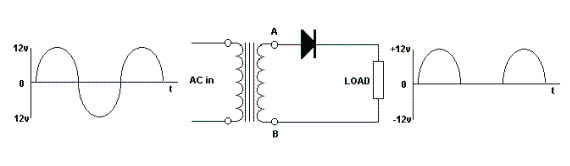Electronic Circuits and Tutorials
Tutorials
Dictionaries
Projects
Discover
Home > Electronics Tutorial > The Diode Tutorial > Half Wave Rectifier Tutorial
The Diode - Electronic Tutorials

# Half Wave Rectifier TutorialThe voltage at point A does the opposite of that at point B. When A is increasing in a positive direction, B is increasing in a negative direction. It is rather like the two ends of a see-saw.

During the first half cycle of the waveform shown on the left, A is positive and B is negative. The diode is forward biased and current flows around the circuit formed by the diode, the transformer winding and the load.

Since the current through the load, and the voltage across the load are in the same proportions, then the voltage across the load is as shown in the right hand diagram, during the first half cycle.

During the second half cycle, A and the anode are negative, B and  the cathode are positive. The diode is reverse biased and no current flows. This is indicated by the horizontal line in the right hand diagram.

The diode only conducts on every other half cycle. There is one pulse for every cycle in. i.e 50 pulses per second (in the UK).

The diode only conducts during half the cycle. Hence, HALF-WAVE RECTIFICATION.

The rectified voltage is DC (it is always positive in value). However, it is not a steady DC but PULSATING DC. It needs to be smoothed before it becomes useful. If the diode is reversed then the output voltage is negative.